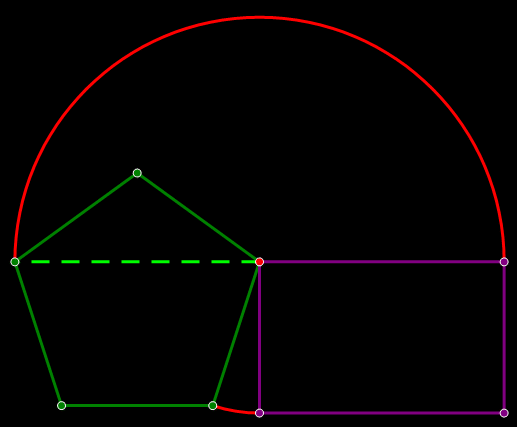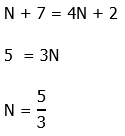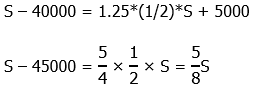offers hundreds of practice questions and video explanations. Go there now.

Intro to GMAT Word Problems, Part 1: Translating from Word to Math

First, four GMAT practice word problems.

1) Seven more than a number is 2 more than four times the number.  What is the number?

(A) 1

(B) 2/3

(C) 3/5

(D) 4/7

(E) 5/3

2) If \$40,000 less than John’s salary is \$5,000 more than 25% more than half his salary, then what is John’s salary?

(A) \$80,000

(B) \$100,000

(C) \$120,000

(D) \$135,000

(E) \$140,000

3) Twice a number is 3 times the square of the number less than one.  If the number is positive, what is the value of the number?

(A) 1

(B) 1/2

(C) 1/3

(D) 2/3

(E) 3/2

4) The original price of an item is discounted 20%. A customer buys the item at this discounted price using a \$20-off coupon.  There is no tax on the item, and this was the only item the customer bought.  If the customer paid \$1.90 more than half the original price of the item, what was the original price of the item?

(A) \$61

(B) \$65

(C) \$67.40

(D) \$70

(E) \$73

GMAT word problems

It’s one thing to understand algebra in the abstract, and quite another to think about where the rubber meets the road.  The reason human beings created algebra was to solve problems about real world situations, and the GMAT loves asking math problems about numbers and about real world situations, a.k.a. word problems!  Even folks who can do algebra in the abstract sometimes find word problems challenging.  In this blog and the next, we present a rough-and-ready guide to what you need about word problems.

Translating from Words to Math

Suppose we have the following sentence in a word problem:

“Three-fifths of x is 14 less than twice y squared.”

How do we change words to math?  Here’s a quick guide

1) the verb “is/are” is the equivalent of an equal sign; the equal sign in an equation is, in terms of “mathematical grammar,” the equivalent of a verb in a sentence.  Every sentence has a verb and every equation has an equal sign.

2) The word “of” means multiply (often used with fractions and percents).  Ex. “26% of x” means (0.26x)

3) The words “more than” or “greater than” mean addition.  Ex. “5 greater than x” means (x + 5) and “7 more than y” means (y + 7)

4) The words “less than” means subtraction.  Ex. “8 less than Q” means (Q – 8).  Notice that the first element is always subtracted: in other words, “J less than K” means (K – J).

With that in mind, let’s go back to the sentence from the hypothetical problem above.

“three fifths of x” means [(3/5)*x]

“is” marks the location of the equal sign

“twice y squared” means 2(y^2)

“14 less than twice y squared” means 2(y^2) – 14

Altogether, the equation we get is:Using this strategy, it’s straightforward to translate from a verbal statement about numbers to an equation.

Other strategies

When all the answer choices are numerical, one further strategy we have at our disposal is backsolving.  Using this strategy, we can pick one answer, plug it into the problem, and see whether it works.  If this choice is too big or too small, it guides us in what other answer choices to eliminate.  Typically, we would start with answer choice (C), but if another answer choice is a particularly convenient choice, then we would start there.

Summary

If the strategies discussed here gave you any insights, you may want to give the problems above another look before turning to the solutions below.  Look for the second article on Assigning Variables in Word Problems.Explanations to Practice Problems

1) Translate this one step at a time.  Let N be the number we seek.

“seven more than a number” = N + 7

The “is” is the equal sign.

“two more than four times the number” = 4N + 22) We will say that S is John’s salary.  “\$40,000 less than John’s salary” is (S – 40000).  The second part is tricky: “25% more than half his salary” is (1.25*(1/2)*S), so “\$5,000 more than 25% more than half his salary” would be that plus 5000.  We can write the whole first part of the prompt sentence asMultiply both sides by 8 (we don’t have to perform the numerical multiplication yet)

8S – 8*45000 = 5S

3S = 8*45000

S = 8*15000 = 4*30000 = 120,000

Notice the use of the doubling and halving trick to perform the multiplication in the last line.  John’s salary must be \$120,000.

3) Call the number x.  Of course, “twice the number” equals 2x.  The part after the word “is” can be tricky.  Remember that the information of the form “J less than K” takes the mathematical form (K – J), in which the first part is the part that’s subtracted.  What we have here is “3 times the square of the number less than one.”  That would be one minus 3 times x squared. Now we can translate that entire first sentence of the prompt into math:This is a quadratic.  We need to get all the terms on one side, equal to zero, and then factor.Because the prompt tells us that the number must be positive, we can reject the negative root.  The number must be +1/3.

4)  We will show two solutions for this: (i) backsolving, and (ii) the full algebraic solution.

For the backsolving solution, notice that (C) is an ugly number.  (B) and (D) are nicer numbers.  Let’s start with (D).

Original price = \$70

10% of price = \$7, so

20% of price = \$14.

After discount, the price is 70 – 14 = \$56.  The customer then uses a \$20-off coupon, so this customer pays \$36.

How does this price compare to half the original price?  Well, half the original was \$35, so the customer paid exactly \$1 more than half the original price.

First of all, we know that answer choice (D) does not work.  We know we need a bigger difference, so we need a bigger price.  The only price bigger is (E).  This must be the answer.

Now, a full algebra solution.  Let the original price be P.  Then, 20% off would be 0.8*P.  Then, if we subtract \$20, that’s (0.8*P – 20).  That is the price the customer paid, which equals “\$1.90 more than half the original price,” or (0.5P + 1.9).  We will set these equal.

08*P – 20 = 0.5*P + 1.9

0.3*P = 21.9

3P = 219

P = 73

The original price was \$73.  Answer = (E)